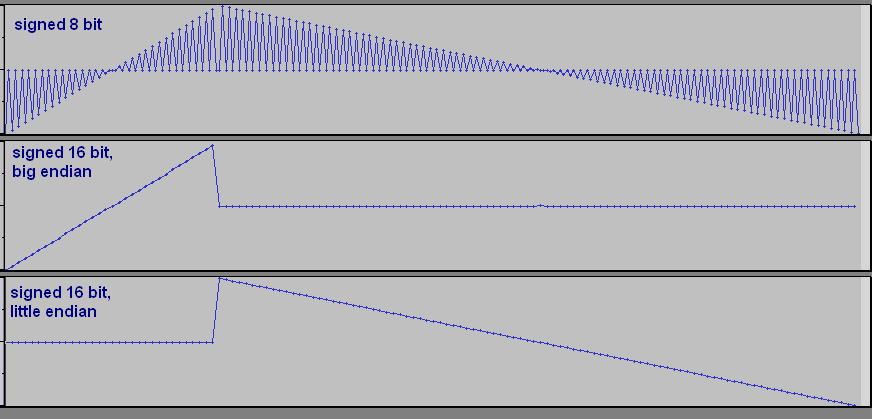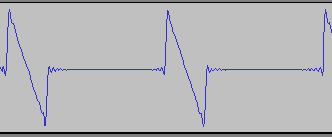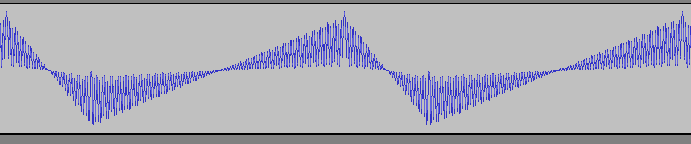# Pitch

Part of OrgMaker Notes

## Results

The OrgMaker playing routines store a wave for each of the 100 melodic instruments. Each wave has 256 points that store one period of the instrument's waveform. Each point is an 8-bit signed value.

The source waveform is transformed by skipping and/or duplicating points to create an intermediate waveform. The intermediate waveform is then played at a certain point frequency to produce sound.

There is one intermediate waveform production pattern used for each OrgMaker octave as follows:

OrgMaker
octave
source points used in
intermediate waveform
period size of
intermediate waveform
0every source point is used 4 times256 * 4 =1024 points
1every source point is used 2 times256 * 2 =512 points
2every other source point is used 2 times256 points
3every 4th source point is used 2 times256 / 2 =128 points
4every 8th source point is used 2 times256 / 4 =64 points
5every 16th source point is used 2 times256 / 8 =32 points
6every 32nd source point is used 2 times256 / 16 =16 points
7every 64th source point is used 2 times256 / 32 =8 points

In all octaves, the intermediate waveform is played at a point frequency for each pitch class as follows:

pitch classpoint frequency
(points per second)
C33408 + (f-1000)
C#35584 + (f-1000)
D37632 + (f-1000)
D#39808 + (f-1000)
E42112 + (f-1000)
F44672 + (f-1000)
F#47488 + (f-1000)
G50048 + (f-1000)
G#52992 + (f-1000)
A56320 + (f-1000)
A#59648 + (f-1000)
B63232 + (f-1000)

f is the Freq number used for the track (range 100 to 1900, default 1000). It acts as a pitch bend, but because it shifts the point frequency by a constant amount, this results in a different pitch shift in cents for each pitch class.

To calculate the pitch frequency (periods per second), divide the point frequency by the period size. For example, for OrgMaker octave 3 and Freq number 1000 you get the following:

OrgMaker pitchpoint frequency
(points per second)
/ period size
(points)
= pitch frequency
(periods per second)
C333408/ 128= 261
C#335584/ 128= 278
D337632/ 128= 294
D#339808/ 128= 311
E342112/ 128= 329
F344672/ 128= 349
F#347488/ 128= 371
G350048/ 128= 391
G#352992/ 128= 414
A356320/ 128= 440
A#359648/ 128= 466
B363232/ 128= 494

## Testing procedure

### Wave data format

When you examine the OrgMaker wave data in a resource editor you can see it is 25600 bytes long. Looking at the data you can see transitions between \$00 and \$FF, which quickly suggest the data is using a signed format. However, it's not obvious if the wave data is 8-bit or 16-bit. Because the data is almost always gradually increasing or gradually decreasing, interpreting the bytes as 8-bit or 16-bit values yeilds similar looking waveforms. Either way, when you plot the signed values, each group of 256 bytes appears to be one period of a waveform, one wave for each of the 100 melodic instruments.

To test what format is used, I used a hex editor to overwrite a wave pattern in OrgMaker with a special test wave. There are three possible data formats I wanted to test for: signed 8-bit, signed 16-bit little endian, and signed 16-bit big endian. The test wave data I came up with alternates between zero bytes and ascending or descending values.

Here is the raw test wave data: test-wave.bin.

Here is what the test wave looks like when you import the raw data into Audacity using the three possible formats.In the ascending section, the first byte of each pair is the ascending value and the second byte of each pair is always zero. In the descending section, the first byte of each pair is always zero, and the second byte of each pair is the descending value.

I made the ascending section take up 1/4 of the wave, and the descending section take up 3/4 of the wave. I did this so I could tell if the values were inverted. For example, if you see a short descending section in the output wave, then you know it came from the short ascending source data.

After I inserted the test wave data into OrgMaker, I played test notes in OrgMaker while recording in Audacity. I expected the output waveform recorded in Audacity to look like one of the waves above.

Note: When making the test recordings, I used Basic hardware acceleration and Good (Standard) sample rate conversion quality. (See Audio Performance settings.)

Here is a recording of a test note from OrgMaker octave 3:Note that the values are inverted: The short ascending section in the source data became a short descending section in the output wave. The long descending section in the source data became close to zero in the output, suggesting OrgMaker is interpreting the source data as signed 16-bit big endian values.

However, if you record a test note from OrgMaker octave 1, you see a different output wave:Again the output values are inverted, the lowest source value is the highest point in the output wave. However, this output wave bounces to and from zero, suggesting OrgMaker is interpreting the source data as signed 8-bit values.

These conflicting test results eventually make sense after working through the other tests below.

### Wave playback rate

OrgMaker uses DirectSound to play audio. OllyDbg doesn't show DirectSound function names automatically. If you download the DirectSound SDK, it has a debug folder that contains a debug version of dsound.dll and dsound.pdb. If you copy these files to the same folder as the OrgMaker executable you're debugging, OllyDbg will show DirectSound function names. However, the function names only appear right before the function is called, so you have to set a breakpoint on a function call instruction, start the program, then when the breakpoint is reached, you can see what DirectSound function name it might be.

Browsing the DirectSound documentation from Microsoft, I suspected the SetFrequency method is what OrgMaker uses to set the playback rate of the wave data. However, because OllyDbg only shows the DirectSound function names right before the function is called, saving the disassembly as text and searching it for "SetFrequency" won't find anything. I needed to find some other way to find potential SetFrequency calls.

According to the Org2XM README file, the Freq number in OrgMaker is used to modify the playback rate of the wave data. The Freq number has a range of 100 to 1900, but it is modified by subtracting 1000 so it ends up having an effect of -900 to +900.

I decided to look for places where a subtraction by 1000 is preformed to see if there's a SetFrequency call nearby. I saved OllyDbg's entire disassembly of OrgMaker to a text file, then searched for any instances of 18FC, the little-endian signed hex value for -1000. I found it exactly once.

Note: I used the English translation of OrgMaker version 2. If you use this process with another version, the addresses may be different.

```0040AC75   8D8410 18FCFFFF   LEA EAX,DWORD PTR DS:[EAX+EDX-3E8]
0040AC7C   50                PUSH EAX
0040AC7D   51                PUSH ECX
0040AC7E   FF55 44           CALL DWORD PTR SS:[EBP+44]
```

To confirm if the call is to SetFrequency do the following. Set a breakpoint at 0040AC7E and start the program. In OrgMaker, click one of the piano keys to make a OrgMaker play a note. The breakpoint is reached and the function name shows up as

```DSOUND.?SetFrequency@?\$CImpDirectSoundBuffer@VCDirectSoundPrimaryBuffer@@@@UAGJK@Z
```

Some additional testing reveals the function is called 16 times before every melodic note OrgMaker plays. I don't know the exact reason why. The value of EAX changes on some passes through the loop. On the 16th call, the final desired playback rate is contained in EAX.

I used a conditional log breakpoint to log the values of EAX. Then I clicked on a piano key in OrgMaker and wrote down the last EAX value used, the playback frequency used for that note.

The interesting result is the same playback rates are used for every OrgMaker octave. If you start with C and go up, the playback rate gets faster each pitch. When you reach the C in the next octave, the playback rate returns to the same rate as the previous C. In other words, each pitch class has the same playback rate no matter what octave it's in.

### Intermediate waveform

The only way the playback rates could produce pitches in the correct octave was if a wave with half as many points was played for each higher octave, and a wave with twice as many points was played for each lower octave. If every other point is skipped when going to a higher octave, this could help explain the different results seen in the wave format test.

I didn't attempt to look for code in the assembly to explain if and how an intermediate waveform is used. Instead, I just made more test waves with zeros or random noise in values I suspected were dropped, and appropriate wave values in values I suspected were kept. I played around with it until I found a matching pattern of what points were kept and what points were discarded.

Because of this intermediate waveform usage, my original test wave to test the format of the source data wasn't getting all of its points used in the higher octaves. In OrgMaker octaves 0 and 1 all of the source points are used, so that shows the wave data is 8-bit signed.